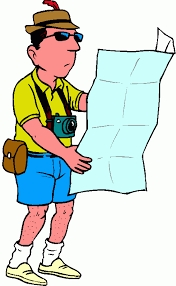# Tourist route

How long is the tourist route when tourists crossed four-sevenths of the way on foot, crossed the bus twice less than on foot, and passed the last 14 kilometers by boat?Did you find an error or inaccuracy? Feel free to write us. Thank you!Tips to related online calculators
Need help to calculate sum, simplify or multiply fractions? Try our fraction calculator.
Do you have a linear equation or system of equations and looking for its solution? Or do you have a quadratic equation?
Do you want to convert length units?